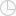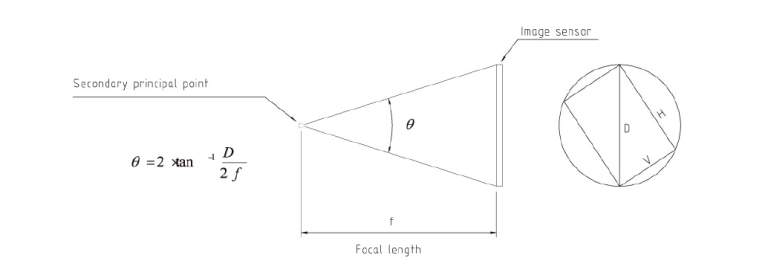## About Angle of View, Aperture (F No.)Time:08 Jul 2014

Angle of View

The angle formed by the 2 line from the secondary principal point to the edges or corners of image sensor is called the angle of view. Theoretically, the focal length of a lens is fixed regardless of the image size of the CCTV camera. Conversely, the angle of view varies according to the change of image size. For a certain image size, the angle of view will increase when the focal length becomes shorter. The focal lengths in the catalog are nominal and the angles of view calculated by the formula referring to the focal length are approximate.Aperture (F No.)

Aperture is an index for the amount of light that passes through a lens.  The value of the aperture is represented by the F No., the smaller the F number, the greater the amount of light, and the brighter the image generated by the lens.  The F No. is inversely proportional to the entrance pupil diameter of the lens and directly proportional to the focal length.  Its formula is as follows: F No. = f / D (f: focal length, D = Entrance pupil diameter)

+86 13570283679
sales@zlkc.com.cn

ZLKC LENS | Machine Vision LENS CCTV Camera lens Industrial lens FA/ITS lens